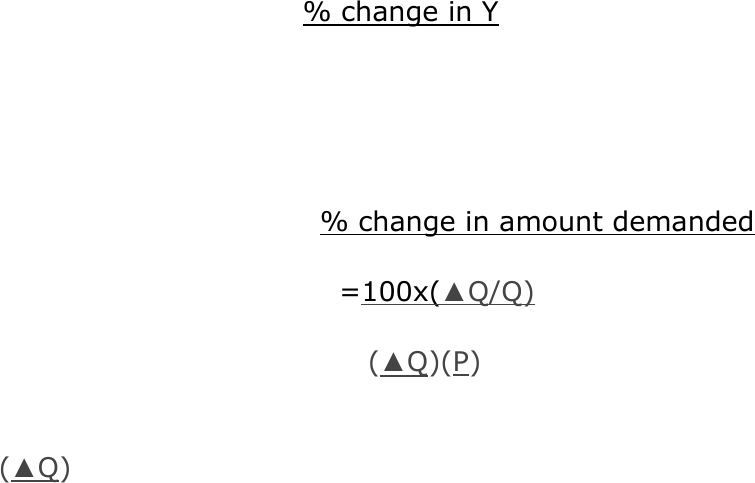Class Notes (1,100,000)
CA (650,000)
U of G (30,000)
REAL (100)
Lecture 4

# REAL 1820 Lecture Notes - Lecture 4: Inverse Demand Function, Demand Curve, Xu

Department
Real Estate and Housing
Course Code
REAL 1820
Professor
Emma Allen- Vercoe
Lecture
4

This preview shows pages 1-3. to view the full 58 pages of the document.
Econ Notes for Final- 2310
Chapter 2
Demand curve shows how much buyers of the product want
to buy at each possible price, holding fixd all other factors that
affect demand
Factors that affect the demand for a product include
population growth consumer tastes, incomes, the price of
other products and government taxes or regulations. a
change in any of these factors will cause the entire
demand curve to shift
A change in price results in a shift along the demand
curve.
Substitutes two products that if all else is equal, an
increase in the price of one of the products cases buyers to
demand more of the other product (eg potatoes and corn)
Complements two products that if all else is equal, an
increase in the price of one of the products causes consumers
to demand less of the other product. (eg butter and corn)
Demand function describes the amount of the product that
is demanded for each possible comination of its price and other
factors
Q= D(Price, other factors)
Q = 14 2P
Inverse demand function how much the firm must charge
to sell any given quantity of its product
P= P(Quantity Demanded)
P= 7 Q/2

Only pages 1-3 are available for preview. Some parts have been intentionally blurred.

Supply Curve shows how much sellers of a product want to
sell at each possible price holding fixed all other factors that
affect supply
Factors that affect supply of a product include
technology, the price of inputs the price tof other possible
outputs and government taxes or regulations. A change
in one of these factors will result in the supply cure
shifting
A change in price will cause movement along the supply
curve
Supply function describes the amount of the prodct that is
supplied for each possible combination of it’s price and other
factors
Q = S(Price, Other factors)
Q= 5p 6
Equilibrium price - the price at which the amounts supplied
and demanded are equal graphically it is the point where the
demand curve and supply curve meet.
When there is an increase in demand (demand curve shift
to the right) Price will increase and quantity will increase
When there is a decrease in demand (demand curve shift
to the left) Price will decrease and quantity will decrease
When there is an increase in supply (supply cure shift to
the right) price will decrease and quantity will increase
When there is a decrease in supply (supply curve shift to
the left) price will increase and quantity will decrease.
When demand and supply increase, price is unknown and
quantity rises
When demand and supply decrease, price is unknown and
quantity falls

Only pages 1-3 are available for preview. Some parts have been intentionally blurred.When demand increases and supply decreases, price rises
and quantity is unknown
When demand dicreases and supply increases price falls
and quantity is unkown
Elasticity the measure of the responsiveness of one ariable
to changes in another variable
E= % change in Y
% change in X
Price elasticity of demand change in the amount
demanded for each percent increase in the price
E(D)= % change in amount demanded
%change in price
=100x(Q/Q)
100x(P/P)
= (Q)(P)
(P)(Q)
(Q) = change in the amount demanded for each dollar that
the price increases, it
(P) can also be refered to as B
E(D)= -B(P/Q)
Elastic when the elasticity of demand is less than negative
one. The percentage change in amount demanded is larger
than the percentage change in price.
Inelastic when the elasticity of demand is greater than -1
but smaller than 0. Percentage change in the amount
demanded is smaller than thepercentage change in price
Perfectly elastic when the demand curve is horizantal so
the demand equals negative infinity